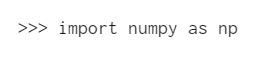top of pageSearch

# What is NumPy?

A NumPy stands for Numerical Python. NumPy is one of the most powerful python libraries. It was created by Travis Oliphant in 2005. It a distributed, volunteer, open-source project.

NumPy is a Python library and is written partially in Python, but most of the parts that require fast computation are written in C or C++.

It provides a high-performance multidimensional array object and tools for working with these arrays. The array object in NumPy is called ndarray, it provides a lot of supporting functions that make working with ndarray very easy. Arrays are very frequently used in data science, where speed and resources are very important.

NumPy can be imported into the notebook usingIt is the fundamental package for scientific computing with Python. It contains various features including these important ones:

• A powerful N-dimensional array object

• Tools for integrating C/C++ and Fortran code

• Useful linear algebra, Fourier transform, and random number capabilities

It has a lot of built-in functions. A full list would be too long but suffice to say there are functions for financial calculations, indexing, linear algebra, math functions, polynomials, random sampling, statistics, binary, logic, sorting, searching, and string operations.

NumPy arrays can be initialized from nested Python lists, and access elements using square brackets:NumPy also provides many functions to create arrays:Some of the important attributes of a NumPy object are:

1. Ndim: displays the dimension of the array

2. Shape: returns a tuple of integers indicating the size of the array

3. Size: returns the total number of elements in the NumPy array

4. Dtype: returns the type of elements in the array, i.e., int64, character

5. Itemsize: returns the size in bytes of each item

6. Reshape: Reshapes the NumPy array

NumPy array elements can be accessed using indexing. Below are some of the useful examples:

· A[2:5] will print items 2 to 4. Index in NumPy arrays starts from 0

· A[2::2] will print items 2 to end skipping 2 items

· A[::-1] will print the array in the reverse order

· A[1:] will print from row 1 to end

Note - The source code for NumPy is located at this GitHub repository https://github.com/numpy/numpy

Thank You for visiting!